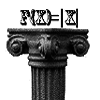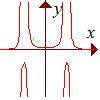# Search by Topic

#### Resources tagged with Functions and their inverses similar to Function Pyramids:

Filter by: Content type:
Age range:
Challenge level:

### There are 13 results

Broad Topics > Functions and Graphs > Functions and their inverses### Function Pyramids

##### Age 16 to 18 Challenge Level:

A function pyramid is a structure where each entry in the pyramid is determined by the two entries below it. Can you figure out how the pyramid is generated?### Inverting Rational Functions

##### Age 16 to 18 Challenge Level:

Consider these questions concerning inverting rational functions### PCDF

##### Age 16 to 18 Challenge Level:

When can a pdf and a cdf coincide?##### Age 16 to 18 Challenge Level:

Decipher a simple code based on the rule C=7P+17 (mod 26) where C is the code for the letter P from the alphabet. Rearrange the formula and use the inverse to decipher automatically.### Double Time

##### Age 16 to 18 Challenge Level:

Crack this code which depends on taking pairs of letters and using two simultaneous relations and modulus arithmetic to encode the message.### Real-life Equations

##### Age 16 to 18 Challenge Level:

Here are several equations from real life. Can you work out which measurements are possible from each equation?### Curve Match

##### Age 16 to 18 Challenge Level:

Which curve is which, and how would you plan a route to pass between them?### Maths Shop Window

##### Age 16 to 18 Challenge Level:

Make a functional window display which will both satisfy the manager and make sense to the shoppers### Modular Fractions

##### Age 16 to 18 Challenge Level:

We only need 7 numbers for modulus (or clock) arithmetic mod 7 including working with fractions. Explore how to divide numbers and write fractions in modulus arithemtic.### Area L

##### Age 16 to 18 Challenge Level:

By sketching a graph of a continuous increasing function, can you prove a useful result about integrals?### Pitchfork

##### Age 16 to 18 Challenge Level:

Plot the graph of x^y = y^x in the first quadrant and explain its properties.### Equation Matcher

##### Age 16 to 18 Challenge Level:

Can you match these equations to these graphs?### Rational Request

##### Age 16 to 18 Challenge Level:

Can you make a curve to match my friend's requirements?